## 5.292. nvisible_from_end

Origin
Constraint

$\mathrm{𝚗𝚟𝚒𝚜𝚒𝚋𝚕𝚎}_\mathrm{𝚏𝚛𝚘𝚖}_\mathrm{𝚎𝚗𝚍}\left(𝙽,\mathrm{𝚅𝙰𝚁𝙸𝙰𝙱𝙻𝙴𝚂}\right)$

Synonyms

$\mathrm{𝚗𝚟𝚒𝚜𝚒𝚋𝚕𝚎}$, $\mathrm{𝚗𝚟𝚒𝚜𝚒𝚋𝚕𝚎}_\mathrm{𝚏𝚛𝚘𝚖}_\mathrm{𝚛𝚒𝚐𝚑𝚝}$.

Arguments
 $𝙽$ $\mathrm{𝚍𝚟𝚊𝚛}$ $\mathrm{𝚅𝙰𝚁𝙸𝙰𝙱𝙻𝙴𝚂}$ $\mathrm{𝚌𝚘𝚕𝚕𝚎𝚌𝚝𝚒𝚘𝚗}\left(\mathrm{𝚟𝚊𝚛}-\mathrm{𝚍𝚟𝚊𝚛}\right)$
Restrictions
 $\mathrm{𝚛𝚎𝚚𝚞𝚒𝚛𝚎𝚍}$$\left(\mathrm{𝚅𝙰𝚁𝙸𝙰𝙱𝙻𝙴𝚂},\mathrm{𝚟𝚊𝚛}\right)$ $𝙽\ge \mathrm{𝚖𝚒𝚗}\left(1,|\mathrm{𝚅𝙰𝚁𝙸𝙰𝙱𝙻𝙴𝚂}|\right)$ $𝙽\le |\mathrm{𝚅𝙰𝚁𝙸𝙰𝙱𝙻𝙴𝚂}|$
Purpose

The ${i}^{\mathrm{𝑡ℎ}}$ $\left(1\le i\le |\mathrm{𝚅𝙰𝚁𝙸𝙰𝙱𝙻𝙴𝚂}|\right)$ variable of the sequence $\mathrm{𝚅𝙰𝚁𝙸𝙰𝙱𝙻𝙴𝚂}$ is visible if and only if all variables after the ${i}^{\mathrm{𝑡ℎ}}$ variable are strictly smaller than the ${i}^{\mathrm{𝑡ℎ}}$ variable itself. $𝙽$ is the total number of visible variables of the sequence of variables $\mathrm{𝚅𝙰𝚁𝙸𝙰𝙱𝙻𝙴𝚂}$.

Example
 $\left(2,〈1,6,2,1,4,8,2〉\right)$ $\left(1,〈3,6,2,1,4,8,8〉\right)$ $\left(7,〈9,8,7,5,4,3,2〉\right)$

The first $\mathrm{𝚗𝚟𝚒𝚜𝚒𝚋𝚕𝚎}_\mathrm{𝚏𝚛𝚘𝚖}_\mathrm{𝚎𝚗𝚍}$ constraint holds since the sequence $1621482$ contains two visible items that respectively correspond to the seventh and sixth items.

Typical
 $|\mathrm{𝚅𝙰𝚁𝙸𝙰𝙱𝙻𝙴𝚂}|>2$ $\mathrm{𝚛𝚊𝚗𝚐𝚎}$$\left(\mathrm{𝚅𝙰𝚁𝙸𝙰𝙱𝙻𝙴𝚂}.\mathrm{𝚟𝚊𝚛}\right)>2$
Symmetry

One and the same constant can be added to the $\mathrm{𝚟𝚊𝚛}$ attribute of all items of $\mathrm{𝚅𝙰𝚁𝙸𝙰𝙱𝙻𝙴𝚂}$.

Arg. properties

Functional dependency: $𝙽$ determined by $\mathrm{𝚅𝙰𝚁𝙸𝙰𝙱𝙻𝙴𝚂}$.

Counting
 Length ($n$) 2 3 4 5 6 7 8 Solutions 9 64 625 7776 117649 2097152 43046721

Number of solutions for $\mathrm{𝚗𝚟𝚒𝚜𝚒𝚋𝚕𝚎}_\mathrm{𝚏𝚛𝚘𝚖}_\mathrm{𝚎𝚗𝚍}$: domains $0..n$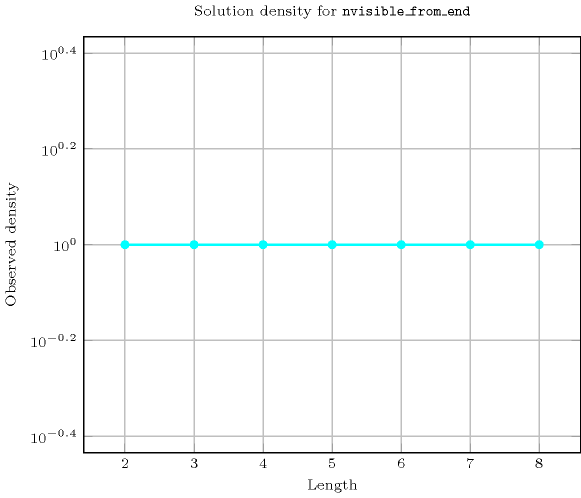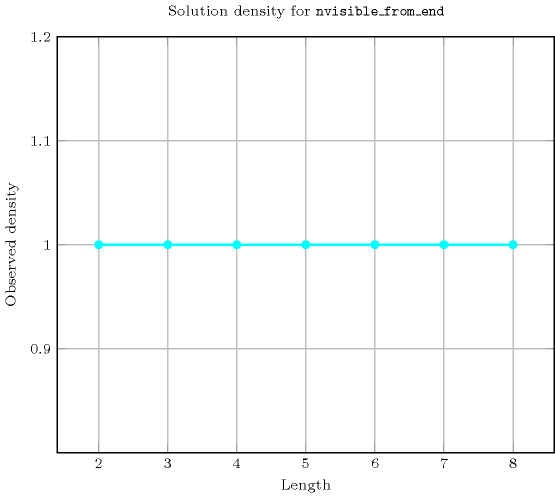Length ($n$)2345678
Total9646257776117649209715243046721
 Parameter value

16302252275290084469648080425
233030536755279488905617238570
3-49016102940058354812780180
4--521060201587604238367
5---642018060661500
6----775646410
7-----81260
8------9

Solution count for $\mathrm{𝚗𝚟𝚒𝚜𝚒𝚋𝚕𝚎}_\mathrm{𝚏𝚛𝚘𝚖}_\mathrm{𝚎𝚗𝚍}$: domains $0..n$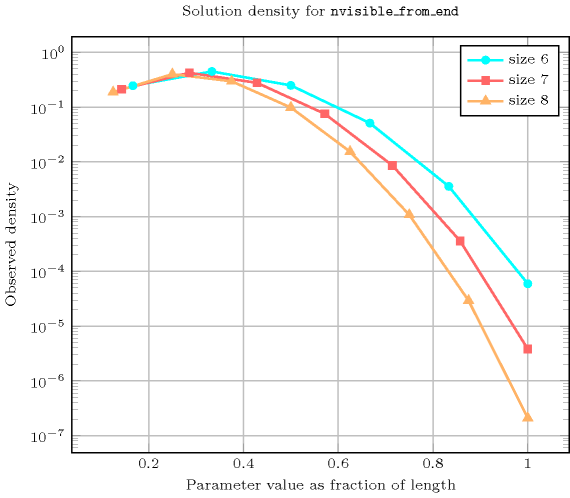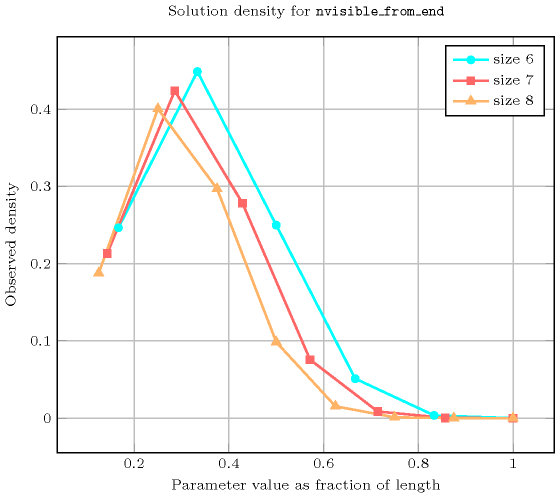related: $\mathrm{𝚗𝚟𝚒𝚜𝚒𝚋𝚕𝚎}_\mathrm{𝚏𝚛𝚘𝚖}_\mathrm{𝚜𝚝𝚊𝚛𝚝}$ (count from the start of the sequence rather than from the end).
Figure 5.292.1 depicts the automaton associated with the $\mathrm{𝚗𝚟𝚒𝚜𝚒𝚋𝚕𝚎}_\mathrm{𝚏𝚛𝚘𝚖}_\mathrm{𝚎𝚗𝚍}$ constraint.
##### Figure 5.292.1. Automaton of the $\mathrm{𝚗𝚟𝚒𝚜𝚒𝚋𝚕𝚎}_\mathrm{𝚏𝚛𝚘𝚖}_\mathrm{𝚎𝚗𝚍}$ constraint with two counters $M$ and $C$, where $M$ records the largest value encountered so far, and $C$ the number of visible values from the right hand side of the sequence ${\mathrm{𝚅𝙰𝚁}}_{1}$, ${\mathrm{𝚅𝙰𝚁}}_{2}$, ..., ${\mathrm{𝚅𝙰𝚁}}_{n}$ (i.e., the sequence ${\mathrm{𝚅𝙰𝚁}}_{n}$, ${\mathrm{𝚅𝙰𝚁}}_{n-1}$, ..., ${\mathrm{𝚅𝙰𝚁}}_{1}$ is passed to the automaton)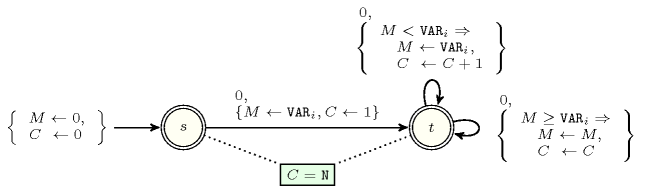##### Figure 5.292.2. Hypergraph of the reformulation corresponding to the automaton (with two counters) of the $\mathrm{𝚗𝚟𝚒𝚜𝚒𝚋𝚕𝚎}_\mathrm{𝚏𝚛𝚘𝚖}_\mathrm{𝚎𝚗𝚍}$ constraint (since all states of the automaton are accepting there is no restriction on the last variable ${Q}_{n}$)